## Sunday, February 23, 2014

### Calculator Art: 2/22/2014

Some calculator art done this week: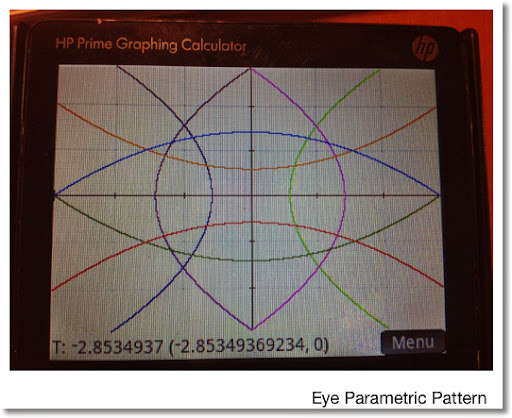Eye Parametric Pattern

HP Prime:

App: Parametric

Range: Autoscale, -2.85349369234 ≤ T ≤ 2.85349369234

Equations:
X1(T) = T
Y1(T) = -0.1736934*T^2 + 1.4142857

X2(T) = T
Y2(T) = -0.1736934*T^2 - 0.587143

X3(T) = T
Y3(T) = -Y1(T)

X4(T) = T
Y4(T) = -Y2(T)

X5(T) = 0.1736934*T^2 - 1.4142857
Y5(T) = T

X6(T) = -X5(T)
Y6(T) = T

X7(T) = 0.17356934*T^2 + 0.5857143
Y7(T) = T

X8(T) = -X7(T)
Y8(T) = T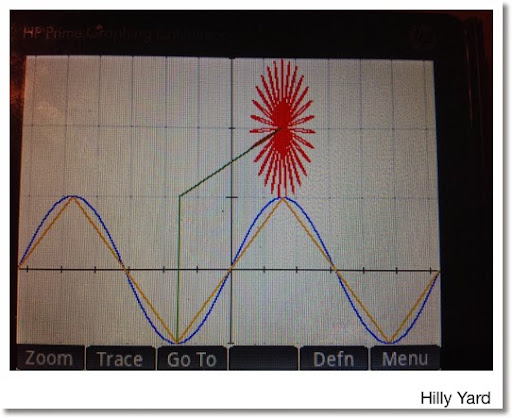Hilly Yard

HP Prime

App: Parametric

Range: -2 π ≤ T ≤ 2 π , Radians Mode

Equations:
X1(T) = T
Y1(T) = PIECEWISE(-2 π ≤ T < -1.5 π, 2T/π + 4,
-1.5 π ≤ T < -0.5 π , -2T/π - 2,
-0.5 π ≤ T < 0.5 π, 2T/π,
0.5 π ≤ T < 1.5 π, -2T/π + 2,
1.5 π ≤ T ≤ 2 π, 2T/π -4)
Color: Orange

X2(T) = T
Y2(T) = sin T
Color: blue

X3(T) = PIECEWISE(-1 ≤ T ≤ 1, -π/2)
Y3(T) = PIECEWISE(-1 ≤ T ≤ 1, T)
Color: Green

X4(T) = PIECEWISE(-π/2 ≤ T ≤ π/2, T)
Y4(T) = PIECEWISE(-π/2 ≤ T ≤ π/2, T/π + 3/2)
Color: Green

X5(T) = 0.15 cos T + π/2
Y5(T) = 0.15 sin T + 2
Color: Red

X6(T) = cos(27*T) sin T + π/2
Y6(T) = cos(27*T) cos T + 2
Color: Red.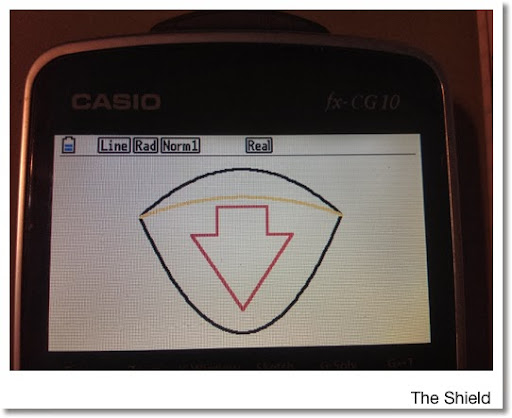The Shield

Casio Prizm

Mode: Graphing

Window:
X = [-3.5, 3.5], Y = [-0.1, 4.5], Range: -2 ≤ T ≤ 2

Equations (Shield):
X1(T) = T
Y1(T) = -0.3125 T^2 + 4.25

X2(T) = T
Y2(T) = 3 T^2 ÷ 4

X3(T) = T
Y3(T) = -0.125 T^2 + 3.5

The arrow was drawn with the FreeLine command.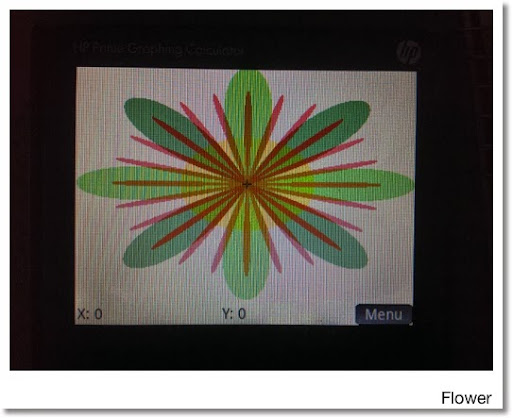Flowers

HP Prime:

Equations:
V1: √(X^2 + Y^2) ≤ 2 cos (24 atan(Y/X))
Color: Red

V2: √(X^2 + Y^2) ≤ 2.5 cos (4 atan(Y/X))
Color: Green

V3: √(X^2 + Y^2) ≤ 2.5 cos (4 (atan(Y/X) - π/4)
Color: Green.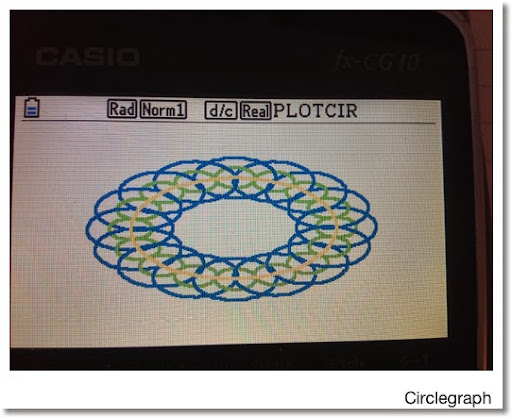Circlegraph

Casio Prizm

Program:
PROGRAM DRAWCIR
Cls
-10 →Xmin
10 → Xmax
-10 → Ymin
10 → Ymax
For 0 → I To 2 π Step π ÷ 8
Plot/Line Color Blue
Circle 5 cos I, 5 sin I, 2
Plot/Line Color Green
Circle 5 cos I, 5 sin I, 1
Next
Plot/Line Color Yellow
Circle 0,0,5

This blog is property of Edward Shore. 2014

## Thursday, February 20, 2014

### A Customized Portable Math Reference

Do you always use a formula that you just can't remember in your head? Or want to carry around a certain amount of facts that are tailored to your applications? Here is a way to do it without having to "lug" around a reference book or having to switch applications on your portable device: portable math cards. The best part is they are 100% customizable. And three to five cards are relatively easy to carry in your pocket. (Yeah I know it is low-tech - I just wanted to try this and see who it works.)

All that is needed are some 3 x 5 index cards, some scissors, and a several plastic baseball card protectors. The index cards are cut to fit the inside of the baseball card protectors. I write on one side of the card, but writing on both side of the cards work.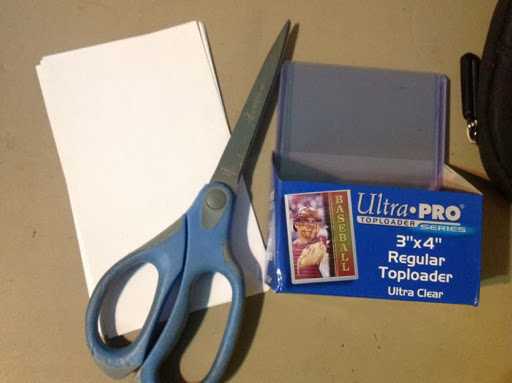The cards contain whatever information you want. For me, I have six cards covering, among other things, basics of electronics, horizontal curves, days between dates formula, some geometric and trigonometric facts.

The point is to create easy to access information without having to either dig through a defense book or apps. Below are pictures of my cards. If you find this idea to be helpful, awesome.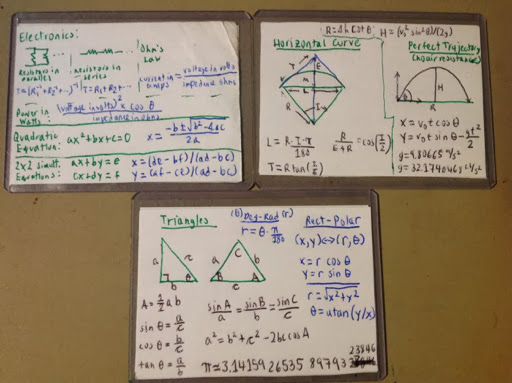Comments and questions are always welcome. Until next time,

Eddie

This blog is property of Edward Shore. 2014

## Sunday, February 16, 2014

### HP Prime: Programming a (Very) Simple Game Part 5

Here it is - a complete simple game.  The objective is to collect dollar signs (coins) to unlock the boundary at the top of the screen to allow access to the goal.  Trees block your progress.  Avoid the purple foes at all times, one touch and it's over!  One purple foe jumps around randomly while two fly horizontal.

Once again, you are controlling the π character.   Press the plus key to quickly exit the game.  This comes in handy if the game ever becomes "unbeatable" (although I tried to knock out all the bugs).

The game features a status bar at the bottom of the screen that shows two things:
*  The number of coins remaining
*  A "LOCKED" indicator that indicates that the boundary is active (red)

This is a culmination of the Simple Game series.  The program is named MOVEM7.

Note: if you are using the emulator, let d1:=1200 and d2:=200.

EXPORT MOVEM7()
BEGIN
// Version 7
// 2014-02-16
// Collect 10 coins to unlock the goal

// A status screen is added on the bottom
// Bottom row is not avialable for play
// This version puts it all together:
// Coins, trees, and enemies

// Game introduction
MSGBOX("Collect all 10 \$ signs to unlock
the goal! Avoid the purple enemies!");

// Clear the screen
RECT();

// Cursor set up
LOCAL A,B,C,D;
A:=150;
B:=216;
C:=150;
D:=216;
TEXTOUT_P("π",A,B,2);

// Draw Coins
LOCAL costr,L2,L3,cx,cy,I,coins;
coins:=10; // counter
L2:=RANDINT(10,0,31)*10;  // x coin
L3:=RANDINT(10,1,17)*12;  // y coin
costr:=CHAR(36);  // \$ sign
FOR I FROM 1 TO 10 DO
cx:=L2(I); cy:=L3(I);
TEXTOUT_P(costr,cx,cy,2,#FF00h);
END;

// Draw Trees
// Trees Setup
LOCAL tstr,L0,L1,S,tx,ty,J;
S:=10;
L0:=RANDINT(S,0,31)*10;   // x tree
L1:=RANDINT(S,1,17)*12;   // y tree
tstr:=CHAR(8857); // tree

// Make sure trees and coins do not
// appear in the same place.
// If there is a conflict, the coin wins.
// Middle
FOR I FROM 2 TO S-1 DO
FOR J FROM 1 TO 10 DO
IF (L0(I)==L2(J)) AND (L1(I)==L3(J)) THEN
// Use CONCAT and SUB
L0:=CONCAT(SUB(L0,1,I-1),SUB(L0,I+1,S));
L1:=CONCAT(SUB(L1,1,I-1),SUB(L1,I+1,S));
// Length of L0 and L1 decrease by 1
S:=S-1;
END;
END;
END;
// Left Side
FOR J FROM 1 TO 10 DO
IF (L0(1)==L2(J)) AND (L1(1)==L3(J)) THEN
L0:=SUB(L0,2,S); L1:=SUB(L1,2,S);
S:=S-1;
END;
END;
// Right Side
FOR J FROM 1 TO 10 DO
IF (L0(S)==L2(J)) AND (L1(S)==L3(J)) THEN
L0:=SUB(L0,1,S-1); L1:=SUB(L1,1,S-1);
S:=S-1;
END;
END;

// Draw the forest
FOR I FROM 1 TO S DO
tx:=L0(I); ty:=L1(I);
TEXTOUT_P(tstr,tx,ty,2,#8000h);
END;

// Ememy Setup
LOCAL estr,d1,d2;
LOCAL ey1A,ey2A,ey3A;
LOCAL ex1A,ex2A,ex3A;
LOCAL ey1B,ey2B,ey3B;
LOCAL ex1B,ex2B,ex3B;

// Delay
d1:=120;d2:=20;
// Enemy 1 jumps to random places
// Enemy 2 flies left to right
// Enemy 3 flies right to left

ex1A:=RANDINT(0,31)*10;
ey1A:=RANDINT(1,17)*12;

ex2A:=−10;
ey2A:=96;

ex3A:=330;
ey3A:=96;

estr:=CHAR(9991); //enemy
TEXTOUT_P(estr,ex1A,ey1A,2,#6000FFh);
TEXTOUT_P(estr,ex2A,ey2A,2,#8000FFh);
TEXTOUT_P(estr,ex3A,ey3A,2,#8000FFh);

// House setup
LOCAL hx,hy,hstr;
hx:=RANDINT(0,31)*10;
hy:=0;
hstr:=CHAR(9820); // castle
TEXTOUT_P(hstr,hx,hy,2,#964B00h);

// Lock setup
LOCAL lock:=1;

// Movement
REPEAT
// Delay Counter
d1:=d1-1; d2:=d2-1;

// Press an Arrow Key
K:=GETKEY;
IF K==7  AND A>0 THEN
C:=A-10; D:=B;
END;
IF K==8  AND A<310 THEN
C:=A+10; D:=B;
END;
IF K==12  AND B<216 THEN
C:=A; D:=B+12;
END;
IF K==2 AND B>0 THEN
C:=A; D:=B-12;
END;

// Move the Enemies
IF d1==0 THEN
d1:=120;

// Enemy 1
ex1B:=RANDINT(0,31)*10;
ey1B:=RANDINT(2,17)*12;
END;

IF d2==0 THEN
d2:=20;

// Enemy 2
ex2B:=ex2A+10;
ey2B:=ey2A;
IF ex2B>330 THEN
ex2B:=−10;
ey2B:=RANDINT(1,17)*12;
END;

// Enemy 3
ex3B:=ex3A-10;
ey3B:=ey3A;
IF ex3B<−10 THEN
ex3B:=330;
ey3B:=RANDINT(1,17)*12;
END;

END;

// Clear previous enemy spot
// Fixed objects will be drawn later
RECT_P(ex1A,ey1A,ex1A+10,ey1A+12);
RECT_P(ex2A,ey2A,ex2A+10,ey2A+12);
RECT_P(ex3A,ey3A,ex3A+10,ey3A+12);

// Draw new enemy positions
TEXTOUT_P(estr,ex1B,ey1B,2,#6000FFh);
ex1A:=ex1B;
ey1A:=ey1B;
TEXTOUT_P(estr,ex2B,ey2B,2,#8000FFh);
ex2A:=ex2B;
ey2A:=ey2B;
TEXTOUT_P(estr,ex3B,ey3B,2,#8000FFh);
ex3A:=ex3B;
ey3A:=ey3B;

// Bump in the boundary?
LOCAL collide:=0;
IF D==hy AND lock==1 THEN
collide:=1;
// No BREAK command because there is no
// FOR loop to break out of
END;

// Ran into a tree?
FOR I FROM 1 TO S DO
IF C==L0(I)  AND D==L1(I) THEN
collide:=1;
BREAK;
// BREAK needed because we have a FOR loop
END;
END;

IF collide==1 THEN
C:=A; D:=B;
END;

// Got hit by the enemy?
LOCAL death;
IF (C==ex1B) AND (D==ey1B) THEN
death:=1;
END;
IF (C==ex2B) AND (D==ey2B) THEN
death:=1;
END;
IF (C==ex3B) AND (D==ey3B) THEN
death:=1;
END;
IF death==1 THEN
MSGBOX("OUCH! :(");
BREAK;
END;

// Collect the coin?
FOR I FROM 1 TO 10 DO
IF C==L2(I)  AND D==L3(I) THEN
L2(I):=-1;
L3(I):=-1;
// Remove coin from play
coins:=coins-1;
BREAK;
END;
END;
// Are all the coins collected?
IF coins==0 THEN lock:=0 END;

// Player
RECT_P(A,B,A+10,B+12);
TEXTOUT_P("π",C,D,2);

// Draw a boundary
IF coins≠0 THEN
LINE_P(0,12,319,12,#FF0000h);
ELSE
// Unlock boundary, all coins collected
LINE_P(0,12,319,12,#FF00h);
END;

// Draw the Goal
TEXTOUT_P(hstr,hx,hy,2,#964B00h);

// Draw the Forest
FOR I FROM 1 TO S DO
tx:=L0(I); ty:=L1(I);
TEXTOUT_P(tstr,tx,ty,2,#8000h);
END;

// Draw the Coins
FOR I FROM 1 TO 10 DO
cx:=L2(I); cy:=L3(I);
// Don't draw at -1
IF L2(I)≠−1 AND L3(I)≠-1 THEN
TEXTOUT_P(costr,cx,cy,2,#FF00h);
END;

END;

// Draw Status Screen
RECT_P(0,217,319,239);
TEXTOUT_P("Coins Left: "+
STRING(IP(coins)),0,228,2);
IF lock==1 THEN
TEXTOUT_P("LOCKED",260,228,2,#FFh);
END;

// Prepare
for the next move
A:=C; B:=D;
// Arrived home?
UNTIL K==50  OR (C==hx AND D==hy);

// Exit Conditions
// Plus Exit
IF K==50 THEN
MSGBOX("Program Terminated.");
END;
// Home Exit
IF C==hx AND D==hy THEN
MSGBOX("Home! :) "+CHAR(9829));
// CHAR(9829) produces a heart
END;

END;

Have fun, and hope you have learned something.

Eddie

This blog is property of Edward Shore, 2014.

### HP Prime: Programming a (Very) Simple Game Part 4

The fourth part of this mini-series will introduce MOVEM6.  In this edition, we will put a condition that is required to be met: collect ten dollar signs (\$), called coins in this game, in order to unlock a barrier at the top of the screen.  Once the barrier is unlocked, the player can get to the goal.

The bottom of the screen will show the number of dollar signs left to collect.

Once again, you are controlling the π character.   Press the plus key to quickly exit the game.

Program:

EXPORT MOVEM6()
BEGIN
// Version 6
// 2014-02-16
// Collect 10 coins to unlock the goal

// A status screen is added on the bottom
// Bottom row is not avialable for play

// Game introduction
MSGBOX("Collect all 10 coins to unlock
the goal!");

// Clear the screen
RECT();

// Cursor set up
LOCAL A,B,C,D;
A:=150;
B:=216;
C:=150;
D:=216;
TEXTOUT_P("π",A,B,2);

// Draw Coins
LOCAL costr,L2,L3,cx,cy,I,coins;
coins:=10; // counter
L2:=RANDINT(10,0,31)*10;
L3:=RANDINT(10,1,18)*12;
costr:=CHAR(36);  // \$ sign
FOR I FROM 1 TO 10 DO
cx:=L2(I); cy:=L3(I);
TEXTOUT_P(costr,cx,cy,2,#FF00h);
END;

// House setup
LOCAL hx,hy,hstr;
hx:=RANDINT(0,31)*10;
hy:=0;
hstr:=CHAR(9820); // castle
TEXTOUT_P(hstr,hx,hy,2,#964B00h);

// Lock setup
LOCAL lock:=1;

// Movement
REPEAT
K:=GETKEY;

// move
IF K==7  AND A>0 THEN
C:=A-10; D:=B;
END;
IF K==8  AND A<310 THEN
C:=A+10; D:=B;
END;
IF K==12  AND B<216 THEN
C:=A; D:=B+12;
END;
IF K==2 AND B>0 THEN
C:=A; D:=B-12;
END;

// Bump in the boundary?
LOCAL collide:=0;
IF D==hy AND lock==1 THEN
collide:=1;
// No BREAK command because there is no
// FOR loop to break out of
END;

IF collide==1 THEN
C:=A; D:=B;
END;

// Collect the coin?
FOR I FROM 1 TO 10 DO
IF C==L2(I)  AND D==L3(I) THEN
L2(I):=-1;
L3(I):=-1;
// Remove coin from play
coins:=coins-1;
IF coins==0 THEN lock:=0 END;
BREAK;
END;
END;

// Player
RECT_P(A,B,A+10,B+12);
TEXTOUT_P("π",C,D,2);

// Draw a boundary
IF coins≠0 THEN
LINE_P(0,12,319,12,#FF0000h);
ELSE
// Unlock boundary, all coins collected
LINE_P(0,12,319,12,#FF00h);
END;

// Draw Static Objects
TEXTOUT_P(hstr,hx,hy,2,#964B00h);

FOR I FROM 1 TO 10 DO
cx:=L2(I); cy:=L3(I);
// Don't draw at -1
IF L2(I)≠−1 AND L3(I)≠-1 THEN
TEXTOUT_P(costr,cx,cy,2,#FF00h);
END;

END;

// Draw Status Screen
RECT_P(0,217,319,239);
TEXTOUT_P(STRING(IP(coins)),0,228,2);

// Prepare
for the next move
A:=C; B:=D;
// Arrived home?
UNTIL K==50  OR (C==hx AND D==hy);
MSGBOX("Home! :)");
END;

Foes, coins, and trees will be combined in the next game.

Eddie

This blog is property of Edward Shore.  2014

## Saturday, February 15, 2014

### Greetings from Santa Barbara (Good Cup): What Makes A Good "Portable" Math Program Language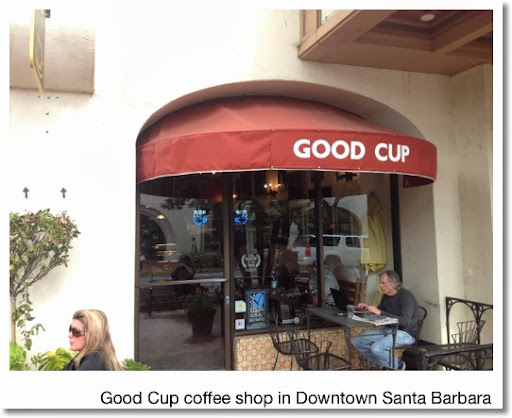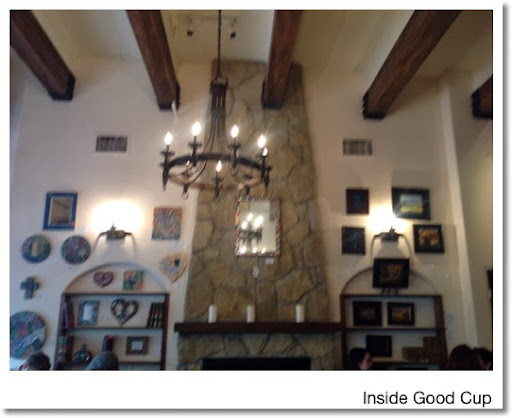I am coming to you today from Santa Barbara and feels so good to get away from the house and work - even it just one day. :). I never thought I would be at a beach in February. I feel for people in the Eastern U.S. who have endured non-stop storms since last autumn.

This blog is being posted from Good Cup, a warm and charming coffee shop in downtown Santa Barbara. I am having their Special Latte - which is a latte made with organic milk. It is very light and taste good - especially after putting some simple syrup in it. Organic food is growing on me. OK, back to the topic at hand...

Portable Programming Calculators

Back in the 1970s and 1980s, programmable calculators were probably at their height where programmable pocket calculators came in the form of the (among other models) 12C, 15C, and 41C from Hewlett Packard, TI-58, TI-66, and TI-68 from Texas Instruments, fx-3650 and fx-6300g from Casio, just to name a few. Since then, portable programming calculators are slowly phased out in favor of graphing calculators, mathematical software, and today portable apps for the iOS and Android.

While it is awesome that we have full-service calculation software on hand today, sometimes all that is needed are quick calculations. Maybe it is during vacation, on a field trip, or doing a project on a long flight.

It is nice to have a portable calculator with programming capabilities. Why?

* It could be the only thing that is reach at the moment.
* The interface usually is more simple.
* If the calculator runs on solar power, like the Casio fx-3650p, that frees up other devices that depend on battery power (either regular or rechargeable).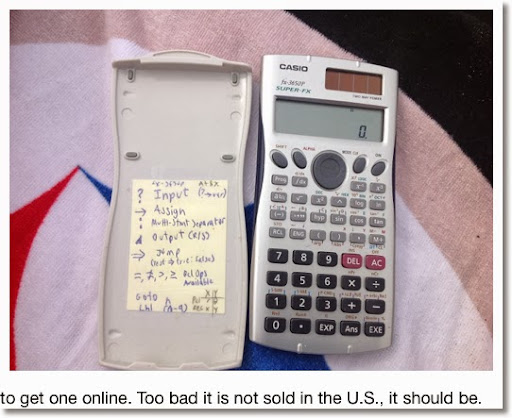Casio fx-3650p

The fx-3650p is a rare combination of a scientific calculator that is both solar-powered and programmable. The memory has four program slots, 360 program steps, and seven storage registers (A,B,C,D,X,Y,M). The M register has the memory arithmetic operations M+ and M-.

The programming language features:
* Prompt for Input
* Display Output
* If-Then-Else structure in the form a Jump command
* Four Boolean comparisons (=, ≠, ≥, >)
* 10 labels for branching.

HP-15C Limited Edition

If a HP-15C LE is desired, it may take luck finding one at a reasonable price. While it is not solar, the HP 15C runs on two long lasting CR2032 batteries. It features RPN keystroke programming, like its super popular financial cousin HP 12C. Capabilities of this landscape calculator include matrices, complex numbers, counter loops, and 448 memory steps.

Other Options (iOS and Android)

There is basically no shortage of programming calculator apps for both iOS and Android devices. A lot of them are \$10 or less, and there are a lot of emulators of older calculators.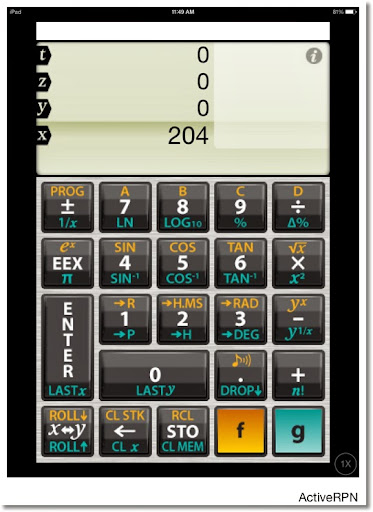Active RPN: (iOS, Android?) This is one example of a programming calculator app. It is one of my favorite non-emulator apps. It is a simple RPN keystroke programming calculator. The memory has 10 numeric registers and 4 program slots of which each can hold up to 80 steps. As far as programming goes, the power is minimal: no comparison tests or loops. (Major drawback).

Below is an HP 67 emulator from Cuveesoft. Very cool to have an emulator available, especially since I never had a physical HP-67 back in the 1970s.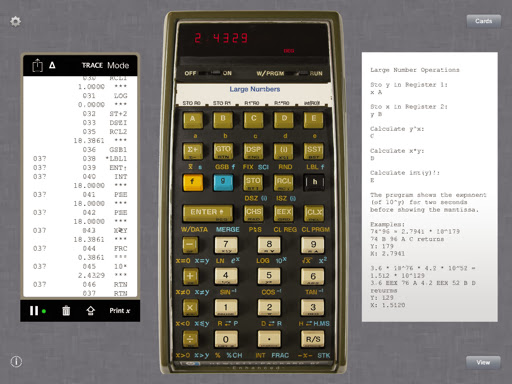Those are just two examples, another is the WP-34, which is an extension of the HP-41C.

What Makes a Good Portable Math Programming Language?

A good portable programmable language can allow for quick numeric calculations for example: program the quadratic formula, calculate the payment of a mortgage, solve probability problems, and store geometric and physics formulas for later use. For me the minimum features the language should have are:

* A definite integral function. Derivatives and sums are a plus.
* Commands to allow for user input.
* Commands to output results and pause the execution of the program.
* Labels for branching, 10 should be good.
* At least 10 memory registers. If alphabetic registers are used, allow for the entire alphabet (A-Z).
* The basic For loop
* Memory should have at least 10 slots with at least 1,000 steps. This will allow long time storage plus some flexibility. If not, a place to carry a small notepad or index cards so we don't have to refinery the wheel each time.

Does this sound like a good list? Is there anything to add or subtract? Let me know in the comments.

Until then, have a great day!

Eddie

This blog is property of Edward Shore. 2014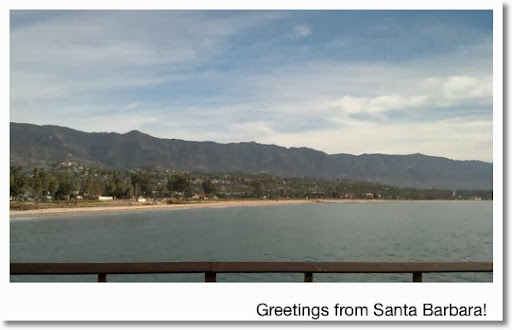## Sunday, February 9, 2014

### HP Prime: Programming a (Very) Simple Game Part 3

The third (and maybe final? TBD) part of this mini-series will introduce MOVEM5.  Unlike stationary trees with no threat in MOVEM4, there are enemies in MOVEM5.  The enemies move up and down.  In this simple game, there are no weapons, you just have to avoid the enemies as you try to head home.  All the enemies are red.  Once touch, and it's game over, so be careful.

Once again, you are controlling the π character.

I left in the "quick exit" key, just press the Plus key to exit at any time.

Code:

EXPORT MOVEM5()
BEGIN
// Version 5
// 2014-02-09
// Get home avoid foes (4)

// Set up the screen
RECT();

// Cursor set up - player
// Two sets of coordinates, original (A,B) and updated (C,D) are

// used
LOCAL A,B,C,D;
A:=0;
B:=60;
C:=0;
D:=60;
TEXTOUT_P("π",A,B,2);

// Ememy Setup
LOCAL estr;
LOCAL ey1A,ey2A,ey3A,ey4A;
LOCAL ex1A,ex2A,ex3A,ex4A;
LOCAL ey1B,ey2B,ey3B,ey4B;
// Set delay paramenters

// This is compensate because the HP Prime acts so fast
// In the emulator I have 4000, 3000, 2000, and 1000 for
// d1, d2, d3, and d4, respectively.
LOCAL d1:=200,d2:=150,d3:=100,d4:=50;

// Initialize position of the enemies
ex1A:=80;
ex2A:=RANDINT(11,17)*10;
ex3A:=RANDINT(20,26)*10;
ex4A:=280;
ey1A:=0;
ey2A:=228;
ey3A:=RANDINT(0,19)*12;
ey4A:=RANDINT(0,19)*12;
estr:=CHAR(9991); //enemy
TEXTOUT_P(estr,ex1A,ey1A,2,#FF0000);
TEXTOUT_P(estr,ex2A,ey2A,2,#FF1000);
TEXTOUT_P(estr,ex3A,ey3A,2,#FF2000);
TEXTOUT_P(estr,ex4A,ey4A,2,#FF3000);

// House setup - our goal
LOCAL hx,hy,hstr;
hx:=RANDINT(29,31)*10;
hy:=RANDINT(0,19)*12;
hstr:=CHAR(9820); // castle
TEXTOUT_P(hstr,hx,hy,2,#964B00h);

// Main loop
REPEAT

// Count down the delay timers
d1:=d1-1;
d2:=d2-1;
d3:=d3-1;
d4:=d4-1;

// Get keystroke from the player
K:=GETKEY;

// Potentially update C and D
IF K==7  AND A>0 THEN
C:=A-10; D:=B;
END;
IF K==8  AND A<310 THEN
C:=A+10; D:=B;
END;
IF K==12  AND B<228 THEN
C:=A; D:=B+12;
END;
IF K==2 AND B>0 THEN
C:=A; D:=B-12;
END;

// Animate the enemies
// Enemy 1: moves up. Wraps around the screen
IF d1==0 THEN

// refresh delay rate - 4000 in the emulator
d1:=200;

// move the enemy
ey1B:=ey1A+12;
IF ey1B>240 THEN
ey1B:=0;
END;
END;

// Draw a blank box of the previous position
RECT_P(ex1A,ey1A,ex1A+10,ex1A+12);

// Draw the enemy at its new location
TEXTOUT_P(estr,ex1A,ey1B,2,#FF0000h);
ey1A:=ey1B;

// Enemy 2 - moving down
IF d2==0 THEN

// Delay rate of 3000 is used in the emulator
d2:=150;
ey2B:=ey2A-12;
IF ey2B<0 THEN
ey2B:=240;
END;
END;
RECT_P(ex2A,ey2A,ex2A+10,ey2A+12);
TEXTOUT_P(estr,ex2A,ey2B,2,#FF0000h);
ey2A:=ey2B;

// Enemy 3 - moving up
IF d3==0 THEN

// Delay rate of 2000 used in the emulator
d3:=100;
ey3B:=ey3A+12;
IF ey3B>240 THEN
ey3B:=0;
END;
END;
RECT_P(ex3A,ey3A,ex3A+10,ex3A+12);
TEXTOUT_P(estr,ex3A,ey3B,2,#FF0000h);
ey3A:=ey3B;

// Enemy 4 - moving down
IF d4==0 THEN

// Delay rate of 1000 used in the emulator
d4:=50;
ey4B:=ey4A-12;
IF ey4B<0 THEN
ey4B:=240;
END;
END;
RECT_P(ex4A,ey4A,ex4A+10,ey4A+12);
TEXTOUT_P(estr,ex4A,ey4B,2,#FF0000h);
ey4A:=ey4B;

// Did we hit the enemy?
LOCAL collide:=0;
IF (C==ex1A) AND (D==ey1B) THEN
collide:=1
END;
IF (C==ex2A) AND (D==ey2B) THEN
collide:=1
END;
IF (C==ex3A) AND (D==ey3B) THEN
collide:=1
END;
IF (C==ex4A) AND (D==ey4B) THEN
collide:=1
END;

// We hit the enemy - not good
IF collide==1 THEN
MSGBOX("OUCH! :(");
KILL;
END;

// Move the player
RECT_P(A,B,A+10,B+12);
TEXTOUT_P("π",C,D,2);

// Draw Static Objects - The House
TEXTOUT_P(hstr,hx,hy,2,#964B00h);

// Prepare for next keystroke
A:=C; B:=D;
// Arrived home? (or hit the Plus key)
UNTIL K==50  OR (C==hx AND D==hy);
MSGBOX("Home! :)");
END;

Until next time,

Eddie

This blog is property of Edward Shore.  2014

### HP Prime: Programming a (Very) Simple Game Part 2

In Part 2, we will actually input some elements that would go into a tile based game.

In this version, called MOVEM4 (I had two beta programs to test and learn things), you are now the Pi (π) character, and your job is to navigate through the forest to the brown castle.
You will not be able to move through the green trees that are scattered through the forest.

There is a blue cloud that buzzes by - that is just for art to make it look like a sunny day.

Like MOVEM, to operate in MOVEM4, use the arrow keys to control your character.  You can always exit by pressing the Plus key.

Here is the code, once again, comments are provided so for those of you who are learning programming, hopefully this helps.  I had a lot of fun doing this.

EXPORT MOVEM4()
BEGIN
// Version 4
// 2014-02-09
// Clear the screen
RECT();

// Cursor set up
// I use two sets of coordinates for the cursor
// A and B for the original and C and D for updated position
LOCAL A,B,C,D;
A:=100;
B:=60;
C:=100;
D:=60;
TEXTOUT_P("π",A,B,2);

// Trees Setup - trees are solid, stopping our player in its tracks
LOCAL tstr;
LOCAL L0,L1,S;
// Random population of a forest
S:=RANDINT(50,100);
L0:=RANDINT(S,0,31)*10;
L1:=RANDINT(S,0,19)*12;
tstr:=CHAR(8857); // tree

LOCAL tx,ty,I;
// Draw the forest
//  #8000h is forest green (RGB(0,128,0))
FOR I FROM 1 TO S DO
tx:=L0(I); ty:=L1(I);
TEXTOUT_P(tstr,tx,ty,2,#8000h);
END;

// House setup - the house is the goal
// Once you get to the house, you win.
//  #964B00h is brown (RGB(150,75,0))
LOCAL hx,hy,hstr;
hx:=RANDINT(0,31)*10;
hy:=RANDINT(0,19)*12;
hstr:=CHAR(9820); // castle
TEXTOUT_P(hstr,hx,hy,2,#964B00h);

// Cloud
// #1E90FF is Dodger Blue (RGB(30,144,255))
LOCAL bx1,by1,bx2,by2,bstr;
bx1:=0;
by1:=RANDINT(0,19)*12;
bstr:=CHAR({10042,10042,10042,
10042,10042}); // cloud
TEXTOUT_P(bstr,bx1,by1,2,#1E90FF);

// Main Loop
REPEAT
// Get a key stroke
K:=GETKEY;

// Update C and D - potential next position
IF K==7  AND A>0 THEN
C:=A-10; D:=B;
END;
IF K==8  AND A<310 THEN
C:=A+10; D:=B;
END;
IF K==12  AND B<228 THEN
C:=A; D:=B+12;
END;
IF K==2 AND B>0 THEN
C:=A; D:=B-12;
END;

// Collision with any of the trees?
LOCAL collide:=0;
FOR I FROM 1 TO S DO
IF C==L0(I)  AND D==L1(I) THEN
collide:=1;
BREAK;
END;
END;

// If yes, you will not move.  Otherwise, you will.
IF collide==1 THEN
C:=A; D:=B;
END;

// Anminate the moving cloud
// In the emulator, I used bx2:==bx1+0.01;
bx2:=bx1+1; by2:=by1;
// If the cloud disappears to the right of the screen,
// it starts over on the left side of the screen
IF bx2>310 THEN
bx2:=0;
by2:=RANDINT(0,19)*12;
END;
RECT_P(bx1,by1,bx1+10,by1+12);
TEXTOUT_P(bstr,bx2,by2,2,#1E90FFh);
bx1:=bx2; by1:=by2;

RECT_P(A,B,A+10,B+12);
TEXTOUT_P("π",C,D,2);

// Draw Static Objects
// The House
TEXTOUT_P(hstr,hx,hy,2,#964B00h);
// The Trees
LOCAL tx,ty,I;
FOR I FROM 1 TO S DO
tx:=L0(I); ty:=L1(I);
TEXTOUT_P(tstr,tx,ty,2,#8000h);
END;
// Prepare for the next keystroke
A:=C; B:=D;
// Loop exits if you arrived home or pressed the Plus Key
UNTIL K==50  OR (C==hx AND D==hy);
MSGBOX("Home! :)");
END;

This may not be the most sufficient way of doing things - but I stress, I am also learning how to do this and thought I share.  Hope this helps, and next time, we face some enemies!

Eddie

This blog is property of Edward Shore - 2014.

### HP Prime: Programming a (Very) Simple Game Part 1

In this and in the next two blog entries will show some of the basics on how to design a simple game.

The first program is called MOVEM.  This really isn't a game, but it is to introduce the GETKEY function on the HP Prime.  The GETKEY gets the last key pressed, while the programmer decides what happens with each key stroke.  Each key will return a key code.

The key codes used are:
2:  up
7:  left
8:  right
12: down
50: plus key

Other common key codes include 30 for Enter.

The HP Prime pixel screen is 320 x 240, with row pixels 0-319 going right, and column pixels 0-239 going down.

The size of the text that is used in MOVEM series is size 2, which means that the characters are 10 pixels long by 12 pixels high.

Comments in the program are followed by two forward slashes.  They don't have to be typed into the program, but they will provide helpful notes.

To run MOVEM, call up MOVEM and move the "M" around the screen using the arrow keys on the arrow pad.  Exit by pressing the Plus (+) button.  Note the code after each arrow movement - your character ("M") will not move beyond the screen's borders.

Code:
EXPORT MOVEM()
BEGIN

// clear the screen
RECT();

// set up the character
A:=100;
B:=60;
TEXTOUT_P("M",A,B,2);

// Start the main loop
REPEAT
K:=GETKEY;

// Move Left
IF K==7  AND A>0 THEN
RECT_P(A,B,A+10,B+12);
A:=A-10;
END;

// Move Right
IF K==8  AND A<320 THEN
RECT_P(A,B,A+10,B+12);
A:=A+10;
END;

// Move Down
IF K==12  AND B<240 THEN
RECT_P(A,B,A+10,B+12);
B:=B+12;
END;

// Move Up
IF K==2 AND B>0 THEN
RECT_P(A,B,A+10,B+12);
B:=B-12;
END;
TEXTOUT_P("M",A,B,2);
// Exit using the Plus Key
UNTIL K==50;

END;

The next blog entry will show how to put a goal and immovable solid objects.

This blog is property of Edward Shore - 2014.

### Set Algebra: Signature of a Permutation: The Easy and Long Way

Hi everyone, I am blogging from a very crowded Coffee Klatch Cafè in San Dimas. Sorry I haven't posted in so long.

Signatures of a Set

In my recent adventures with my calculator, I discovered a function on the HP Prime called signature.

The signature, symbolized as sgn, function returns:

-1 if the permutation has an odd amount of transpositions and
+1 if the permutation has an even amount of transpositions.

It has been a while since I have studied set theory.

When using set theory, we are usually looking at sets like {1}, {1,2}, {1,2,3}, and the like. The basic format is {1,2,3,4...n} where n is a positive integer.

A permutation of a set is just the act of rearranging the set's elements to a different order. For a this type of set, there are n! (n factorial) permutations. For instance, there are 3!, or 6 permutations of the set {1,2,3}.

The permutations of the set {1,2,3} are {1,2,3}, {1,3,2}, {2,1,3}, {2,3,1}, {3,2,1}, and {3,1,2}.

A transposition of a set is just switching two elements.

Example:

From the set {1,2,3,4,5}, a transposition is made when 1 and 5 are switched:
{5, 2, 3, 4, 1}

We can cause another transposition by switching 3 and 4 from the last result:
{5, 2, 4, 3, 1}

Another transposition can be created, let's switch 2 and 4 this time:
{5, 4, 2 , 3, 1}

Determining the signature of each can be determined as follows:

We start out with {1,2,3,4,5}. Nothing has been done, so signature({1,2,3,4,5})=1.

With {5,2,3,4,1}, 1 transposition happened, hence signature({5,2,3,4,1})=-1.

With {5,2,4,3,1}, 2 transpositions took place, and signature({5,2,4,3,1})=1.

Finally 3 transpositions were needed to get {5,4,2,3,1}, so signature({5,4,2,3,1)}=-1.

Do this manually, one should start from the basic permutation {1,2,3,...n}. Simple, but also can cause a lot of work without extensive theory or software.

More information, including way more element mathematics and theory can be found here:

http://en.wikipedia.org/wiki/Parity_of_a_permutation

Have a great day and I will talk to you soon!

Eddie

This blog is property of Edward Shore. 2014

### HP 15C: Error Function and Lower Tail Normal Cumulative Function

HP 15C:   Error Function and Lower Tail Normal Cumulative Function Formulas Used  Error Function  erf(x) = 2 ÷ √π * ∫( e^(-t^2) dt, t = 0 t...